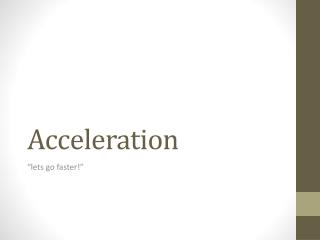DownloadDownload PresentationAcceleration

# Acceleration

Télécharger la présentation## Acceleration

- - - - - - - - - - - - - - - - - - - - - - - - - - - E N D - - - - - - - - - - - - - - - - - - - - - - - - - - -
##### Presentation Transcript

1. Acceleration “lets go faster!”

2. Some background • Before we start acceleration lets review velocity. • Velocity is the change in position over the change in time. • V = Δx / Δt • Velocity unlike speed has a direction. Therefore can be negative.

3. Graphing it • When we graphed my movement back and forth using velocity it showed a zig-zag pattern.

4. Graphing it • When we accelerate we are changing our velocity. • So what do you think the equation is if it deals with changing our velocity? Remember velocity was our change in position.

5. Graphing it In our velocity equation our slope was the change in position vs the change in time. For acceleration the slope is the change in Velocity vs. our change in time. So if the velocity is constant, what is our acceleration. (remember acceleration is the change in velocity)

6. Graphing it • If the slope of position vs time (velocity) is constant then there is no change in velocity therefore no acceleration. • Acceleration can only occur IF there is a change in velocity. • Try graphing it out with me.

7. Example • Sam is testing out his acceleration. He has gone from 0 m/s to 60 m/s in 20 seconds. What is his acceleration?

8. You try • John from rest starts accelerating at 2 m/s2 after 30 seconds what is his final velocity?